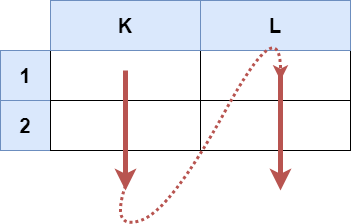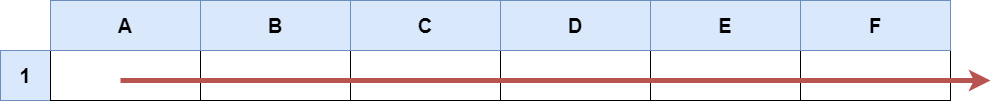| English | 简体中文 |

# 2194. Cells in a Range on an Excel Sheet

## Description

A cell (r, c) of an excel sheet is represented as a string "<col><row>" where:

• <col> denotes the column number c of the cell. It is represented by alphabetical letters.
• For example, the 1st column is denoted by 'A', the 2nd by 'B', the 3rd by 'C', and so on.
• <row> is the row number r of the cell. The rth row is represented by the integer r.

You are given a string s in the format "<col1><row1>:<col2><row2>", where <col1> represents the column c1, <row1> represents the row r1, <col2> represents the column c2, and <row2> represents the row r2, such that r1 <= r2 and c1 <= c2.

Return the list of cells (x, y) such that r1 <= x <= r2 and c1 <= y <= c2. The cells should be represented as strings in the format mentioned above and be sorted in non-decreasing order first by columns and then by rows.

Example 1:Input: s = "K1:L2"
Output: ["K1","K2","L1","L2"]
Explanation:
The above diagram shows the cells which should be present in the list.
The red arrows denote the order in which the cells should be presented.


Example 2:Input: s = "A1:F1"
Output: ["A1","B1","C1","D1","E1","F1"]
Explanation:
The above diagram shows the cells which should be present in the list.
The red arrow denotes the order in which the cells should be presented.


Constraints:

• s.length == 5
• 'A' <= s <= s <= 'Z'
• '1' <= s <= s <= '9'
• s consists of uppercase English letters, digits and ':'.